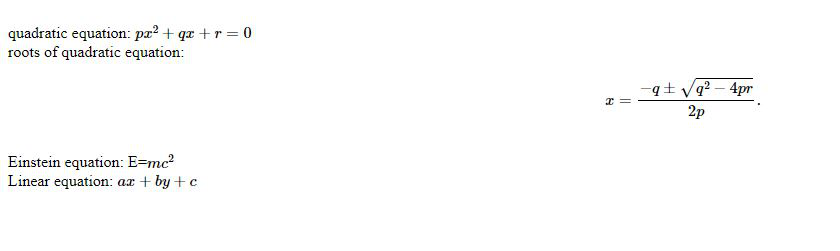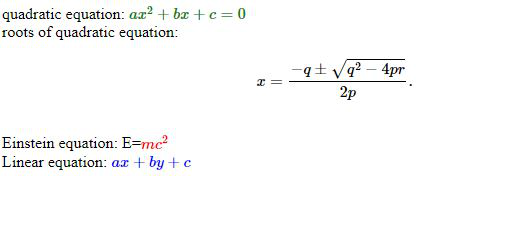Related Articles
Introduction to MathJax
• Last Updated : 15 Jul, 2020

MathJax is a library written in JavaScript, that is used to display mathematical formulas and notations in the browsers quite easily and efficiently. It is an open-source JavaScript display engine for LaTeX, MathML, and AsciiMath notation that works in all modern browsers. MathJax does not require any kind of setup making it easier on the client-side to view it naturally and without any difficulty. This library is built on the top of MathML.

Features:

• Easy to write in the browser and flexible to read.
• Supported in most of the latest versions of the browsers.
• Powerful API Integration.

Installation using a CDN:

<script id="MathJax-script" async
src="https://cdn.jsdelivr.net/npm/mathjax@3/es5/tex-mml-chtml.js">
</script>


Installation in Nodejs:

npm install mathjax

The below examples illustrate the use-cases of MathJax:

Example 1:

## HTML

 <html lang="en">  <head>    <meta charset="UTF-8">    <meta name="viewport" content="width=device-width,                 initial-scale=1.0">    <title>Math jax  <body>          <br />quadratic equation:    $$px^2 + qx + r = 0$$    <br />    roots of quadratic equation:    $$x = {-q \pm \sqrt{q^2-4pr} \over 2p}.$$    <br />    Einstein equation: E=$$mc^2$$    <br />    Linear equation: $$ax+by+c$$          <script id="MathJax-script" async src="https://cdn.jsdelivr.net/npm/mathjax@3/es5/tex-mml-chtml.js">              <script>        MathJax = {            tex: {                inlineMath: [['$', '$'],                             ['\$$', '\$$']]            },            svg: {                fontCache: 'global'            }        };      

Output:Example 2: This example shows the changing of the color of the text.

## HTML

 <html lang="en">  <head>    <meta charset="UTF-8">    <meta name="viewport" content="width=device-width,                 initial-scale=1.0">    <title>Math jax  <body>              <br />quadratic equation:    ${\color{green} ax^2+bx+c=0}$    <br />    roots of quadratic equation:    $$x = {-q \pm \sqrt{q^2-4pr} \over 2p}.$$    <br />          Einstein equation: E=${\color{red} mc^2}$    <br />    Linear equation: ${\color{blue} ax+by+c}$          <script id="MathJax-script" async src="https://cdn.jsdelivr.net/npm/mathjax@3/es5/tex-mml-chtml.js">              <script>        MathJax = {            autoload: {                color: [],                colorV2: ['color']            },            tex: {                inlineMath: [['$', '$'],                         ['\$$', '\$$']]            },            svg: {                fontCache: 'global'            }        };      

Output:My Personal Notes arrow_drop_up